Caffe
caffe::EuclideanLossLayer< Dtype > Class Template Reference

Computes the Euclidean (L2) loss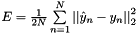for real-valued regression tasks. More...

#include <euclidean_loss_layer.hpp>

Inheritance diagram for caffe::EuclideanLossLayer< Dtype >: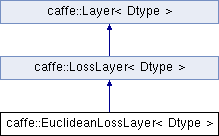## Public Member Functions

EuclideanLossLayer (const LayerParameter &param)

virtual void Reshape (const vector< Blob< Dtype > *> &bottom, const vector< Blob< Dtype > *> &top)
Adjust the shapes of top blobs and internal buffers to accommodate the shapes of the bottom blobs. More...

virtual const char * type () const
Returns the layer type.

virtual bool AllowForceBackward (const int bottom_index) constPublic Member Functions inherited from caffe::LossLayer< Dtype >
LossLayer (const LayerParameter &param)

virtual void LayerSetUp (const vector< Blob< Dtype > *> &bottom, const vector< Blob< Dtype > *> &top)
Does layer-specific setup: your layer should implement this function as well as Reshape. More...

virtual int ExactNumBottomBlobs () const
Returns the exact number of bottom blobs required by the layer, or -1 if no exact number is required. More...

virtual bool AutoTopBlobs () const
For convenience and backwards compatibility, instruct the Net to automatically allocate a single top Blob for LossLayers, into which they output their singleton loss, (even if the user didn't specify one in the prototxt, etc.).

virtual int ExactNumTopBlobs () const
Returns the exact number of top blobs required by the layer, or -1 if no exact number is required. More...Public Member Functions inherited from caffe::Layer< Dtype >
Layer (const LayerParameter &param)

void SetUp (const vector< Blob< Dtype > *> &bottom, const vector< Blob< Dtype > *> &top)
Implements common layer setup functionality. More...

Dtype Forward (const vector< Blob< Dtype > *> &bottom, const vector< Blob< Dtype > *> &top)
Given the bottom blobs, compute the top blobs and the loss. More...

void Backward (const vector< Blob< Dtype > *> &top, const vector< bool > &propagate_down, const vector< Blob< Dtype > *> &bottom)
Given the top blob error gradients, compute the bottom blob error gradients. More...

vector< shared_ptr< Blob< Dtype > > > & blobs ()
Returns the vector of learnable parameter blobs.

const LayerParameter & layer_param () const
Returns the layer parameter.

virtual void ToProto (LayerParameter *param, bool write_diff=false)
Writes the layer parameter to a protocol buffer.

Dtype loss (const int top_index) const
Returns the scalar loss associated with a top blob at a given index.

void set_loss (const int top_index, const Dtype value)
Sets the loss associated with a top blob at a given index.

virtual int MinBottomBlobs () const
Returns the minimum number of bottom blobs required by the layer, or -1 if no minimum number is required. More...

virtual int MaxBottomBlobs () const
Returns the maximum number of bottom blobs required by the layer, or -1 if no maximum number is required. More...

virtual int MinTopBlobs () const
Returns the minimum number of top blobs required by the layer, or -1 if no minimum number is required. More...

virtual int MaxTopBlobs () const
Returns the maximum number of top blobs required by the layer, or -1 if no maximum number is required. More...

virtual bool EqualNumBottomTopBlobs () const
Returns true if the layer requires an equal number of bottom and top blobs. More...

bool param_propagate_down (const int param_id)
Specifies whether the layer should compute gradients w.r.t. a parameter at a particular index given by param_id. More...

void set_param_propagate_down (const int param_id, const bool value)
Sets whether the layer should compute gradients w.r.t. a parameter at a particular index given by param_id.

## Protected Member Functions

virtual void Forward_cpu (const vector< Blob< Dtype > *> &bottom, const vector< Blob< Dtype > *> &top)
Computes the Euclidean (L2) lossfor real-valued regression tasks. More...

virtual void Forward_gpu (const vector< Blob< Dtype > *> &bottom, const vector< Blob< Dtype > *> &top)
Using the GPU device, compute the layer output. Fall back to Forward_cpu() if unavailable.

virtual void Backward_cpu (const vector< Blob< Dtype > *> &top, const vector< bool > &propagate_down, const vector< Blob< Dtype > *> &bottom)
Computes the Euclidean error gradient w.r.t. the inputs. More...

virtual void Backward_gpu (const vector< Blob< Dtype > *> &top, const vector< bool > &propagate_down, const vector< Blob< Dtype > *> &bottom)
Using the GPU device, compute the gradients for any parameters and for the bottom blobs if propagate_down is true. Fall back to Backward_cpu() if unavailable.Protected Member Functions inherited from caffe::Layer< Dtype >
virtual void CheckBlobCounts (const vector< Blob< Dtype > *> &bottom, const vector< Blob< Dtype > *> &top)

void SetLossWeights (const vector< Blob< Dtype > *> &top)

## Protected Attributes

Blob< Dtype > diff_Protected Attributes inherited from caffe::Layer< Dtype >
LayerParameter layer_param_

Phase phase_

vector< shared_ptr< Blob< Dtype > > > blobs_

vector< bool > param_propagate_down_

vector< Dtype > loss_

## Detailed Description

### template<typename Dtype> class caffe::EuclideanLossLayer< Dtype >

Computes the Euclidean (L2) lossfor real-valued regression tasks.

Parameters
 bottom input Blob vector (length 2)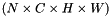the predictionsthe targets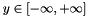top output Blob vector (length 1)the computed Euclidean loss:This can be used for least-squares regression tasks. An InnerProductLayer input to a EuclideanLossLayer exactly formulates a linear least squares regression problem. With non-zero weight decay the problem becomes one of ridge regression – see src/caffe/test/test_sgd_solver.cpp for a concrete example wherein we check that the gradients computed for a Net with exactly this structure match hand-computed gradient formulas for ridge regression.

(Note: Caffe, and SGD in general, is certainly not the best way to solve linear least squares problems! We use it only as an instructive example.)

## ◆ AllowForceBackward()

template<typename Dtype >
 virtual bool caffe::EuclideanLossLayer< Dtype >::AllowForceBackward ( const int bottom_index ) const
inlinevirtual

Unlike most loss layers, in the EuclideanLossLayer we can backpropagate to both inputs – override to return true and always allow force_backward.

Reimplemented from caffe::LossLayer< Dtype >.

## ◆ Backward_cpu()

template<typename Dtype >
 void caffe::EuclideanLossLayer< Dtype >::Backward_cpu ( const vector< Blob< Dtype > *> & top, const vector< bool > & propagate_down, const vector< Blob< Dtype > *> & bottom )
protectedvirtual

Computes the Euclidean error gradient w.r.t. the inputs.

Unlike other children of LossLayer, EuclideanLossLayer can compute gradients with respect to the label inputs bottom (but still only will if propagate_down is set, due to being produced by learnable parameters or if force_backward is set). In fact, this layer is "commutative" – the result is the same regardless of the order of the two bottoms.

Parameters
 top output Blob vector (length 1), providing the error gradient with respect to the outputsThis Blob's diff will simply contain the loss_weight*, asis the coefficient of this layer's outputin the overall Net loss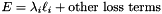; hence. (*Assuming that this top Blob is not used as a bottom (input) by any other layer of the Net.) propagate_down see Layer::Backward. bottom input Blob vector (length 2)the predictions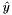; Backward fills their diff with gradientsif propagate_downthe targets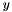; Backward fills their diff with gradientsif propagate_down

Implements caffe::Layer< Dtype >.

## ◆ Forward_cpu()

template<typename Dtype >
 void caffe::EuclideanLossLayer< Dtype >::Forward_cpu ( const vector< Blob< Dtype > *> & bottom, const vector< Blob< Dtype > *> & top )
protectedvirtual

Computes the Euclidean (L2) lossfor real-valued regression tasks.

Parameters
 bottom input Blob vector (length 2)the predictionsthe targetstop output Blob vector (length 1)the computed Euclidean loss:This can be used for least-squares regression tasks. An InnerProductLayer input to a EuclideanLossLayer exactly formulates a linear least squares regression problem. With non-zero weight decay the problem becomes one of ridge regression – see src/caffe/test/test_sgd_solver.cpp for a concrete example wherein we check that the gradients computed for a Net with exactly this structure match hand-computed gradient formulas for ridge regression.

(Note: Caffe, and SGD in general, is certainly not the best way to solve linear least squares problems! We use it only as an instructive example.)

Implements caffe::Layer< Dtype >.

## ◆ Reshape()

template<typename Dtype >
 void caffe::EuclideanLossLayer< Dtype >::Reshape ( const vector< Blob< Dtype > *> & bottom, const vector< Blob< Dtype > *> & top )
virtual

Adjust the shapes of top blobs and internal buffers to accommodate the shapes of the bottom blobs.

Parameters
 bottom the input blobs, with the requested input shapes top the top blobs, which should be reshaped as needed

This method should reshape top blobs as needed according to the shapes of the bottom (input) blobs, as well as reshaping any internal buffers and making any other necessary adjustments so that the layer can accommodate the bottom blobs.

Reimplemented from caffe::LossLayer< Dtype >.

The documentation for this class was generated from the following files: